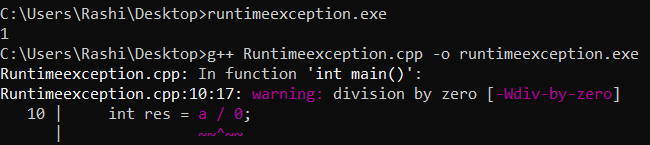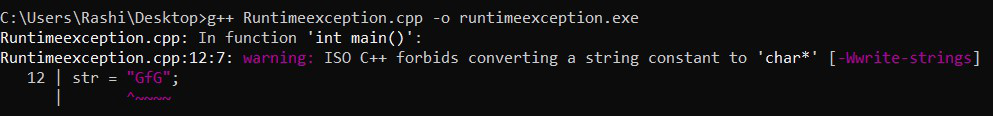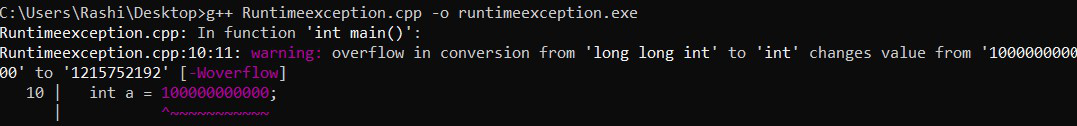Open In App

# C++ Program to Show Runtime Exceptions

A runtime error occurs while the program is running. Because this is not a compilation error, the compilation will be completed successfully. Here, we will learn how to handle runtime exceptions in C++.

There are 5 types of runtime exceptions discussed here:

1. Division by zero.
2. Segmentation faults.
3. Large memory allocation/Large Static Memory Allocation.
4. Type Specifier Error.
5. Invalid memory access during runtime.

Let’s start discussing each of these runtime errors in detail.

## 1. Division By Zero

When we divide an integer value by zero then we get this type of error called division by zero error. It is also called floating-point exceptions (SIGFPE). Below is the C++ program to demonstrate division by zero exception:

## C++

 `// C++ program to demonstrate ``// division by zero exception``#include ``using` `namespace` `std;`` ` `// Driver code``int` `main() ``{``    ``int` `a = 10; ``    ``int` `res = a / 0; ``    ``cout << res;``    ``return` `0;``}`

Output:## 2. Segmentation Faults

In the below code, the line *(str + 1) = ‘n’ tries to write to read-only memory. Below is the C++ program to demonstrate segmentation fault:

## C++

 `// C++ program to demonstrate ``// segmentation fault``#include ``using` `namespace` `std;`` ` `// Driver code``int` `main()``{``  ``char` `*str;``   ` `  ``// Stored in read only part ``  ``// of data segment ``  ``str = ``"GeeksforGeeks"``;    ``   ` `  ``// Trying to modify read only ``  ``// memory``  ``*(str + 1) = ``'n'``;``  ``return` `0;``}`

Output:## 3. Large memory Allocation/Large Static Memory Allocation

In general, any compiler or any language will accept up to 10^8. However, to be on the safe side we generally used up to 10^7. In the below code, the size of the array is more than 10^8 so here we got an error due to large memory allocation. It is also called an abort signal (SIGABRT).

Below is the C++ program to demonstrate large memory allocation runtime exception:

## C++

 `// C++ program to demonstrate ``// large memory allocation``// runtime exception``#include ``using` `namespace` `std;`` ` `// Driver code``int` `main() ``{``  ``int` `a = 100000000000; ``  ``int` `* arr = ``new` `int``[a];``  ``return` `0;``}`

Output:## 4. Type Specifier Error

The below code gives runtime error because here the variable “a” is defined as long long int but in scanf the format specifier used is %d instead of %lld this will cause the error.

Below is the C++ program to demonstrate the type specifier error:

## C++

 `// C++ program to demonstrate ``// type specifier error``#include ``using` `namespace` `std;`` ` `// Driver code``int` `main() ``{``    ``long` `long` `int` `a; ``    ``scanf``(``"%d"``, &a);``    ``return` `0;``}`

Output:

prog.cpp: In function ‘int main()’:

prog.cpp:10:19: warning: format ‘%d’ expects argument of type ‘int*’, but argument 2 has type ‘long long int*’ [-Wformat=]

scanf(“%d”, &a);

^

## 5. Invalid Memory Access During Runtime

In the below code, the array is assigned with a negative index value and this will cause invalid memory access. It will give a garbage value. Below is the C++ program to demonstrate invalid memory access during runtime:

## C++

 `// C++ program to demonstrate ``// invalid memory access during``// runtime``#include ``using` `namespace` `std;`` ` `int` `arr;`` ` `// Driver code``int` `main() ``{``  ``int` `a = arr[-10];``  ``cout << a;``  ``return` `0;``}`

Output

`281923776`